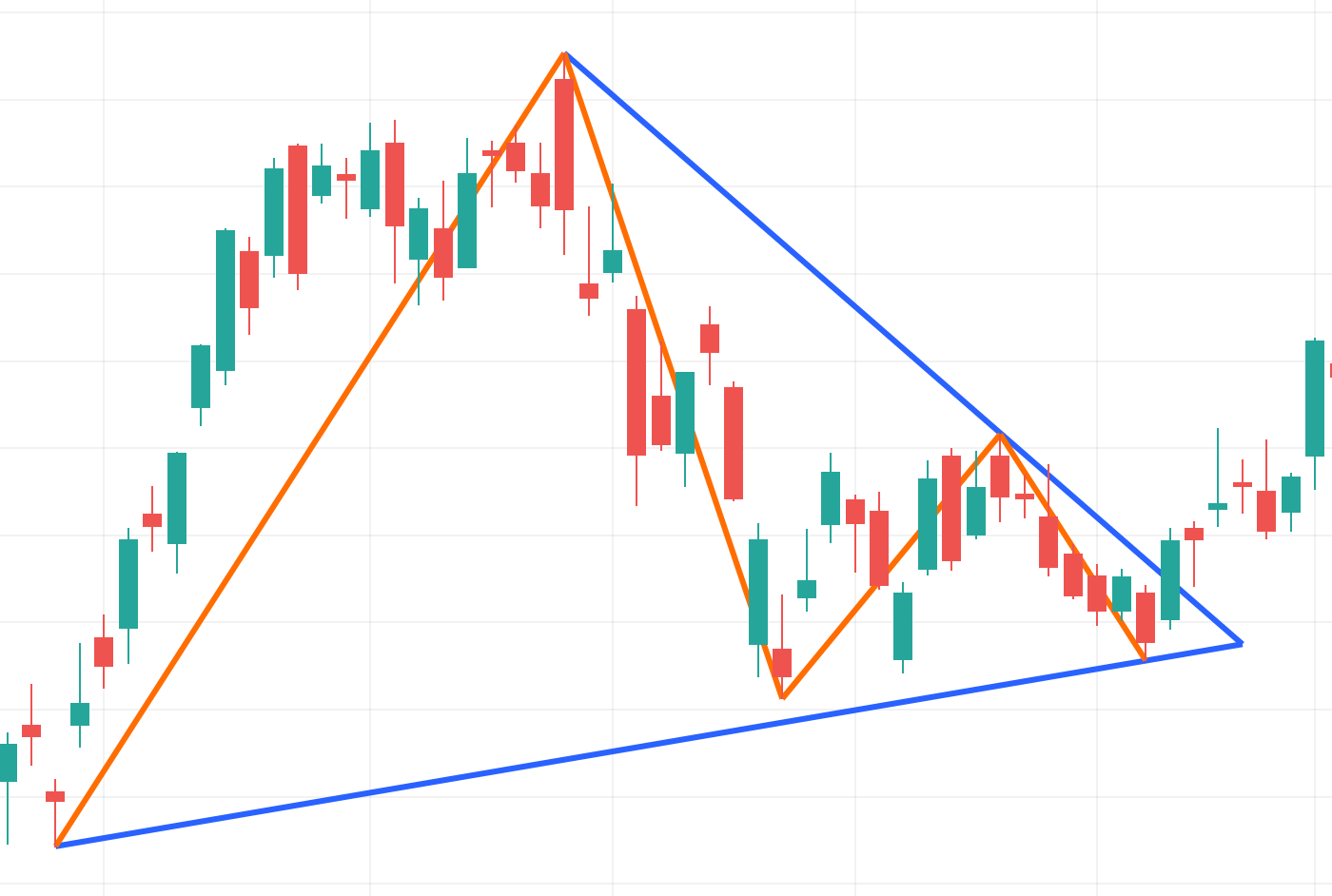Chart Pattern Triangle

A triangle is an indefinite pattern that can mean both a continuation and a reversal of the trend after a consolidation area.The indicator analyzes the last 600 bars in search of matches with a given template of 5 points. The points found by the indicator are tied to the most suitable pivot points. Once the points are found, the indicator checks the pattern rules and the specified maximum permissible deviation.In the interval between points 1 and 5 from the bottom and points 2 and 5 from the top, the price is allowed to cross the triangle lines with ‘high’ and ‘low’, but not with ‘close’ or ‘open’. Of all the found patterns that fit the conditions, the largest non-overlapping triangles are drawn on the chart.

Inputs:

Invert Pattern - Changes the sequence of high and low pivots in the triangle.

Source - Source for the primary search.

Permissible Deviation - The maximum allowable deviation of the midpoint from the triangle line. The deviation is determined by the formula:

Deviation = ((x - x2) * 100) / (x1 - x2), where: